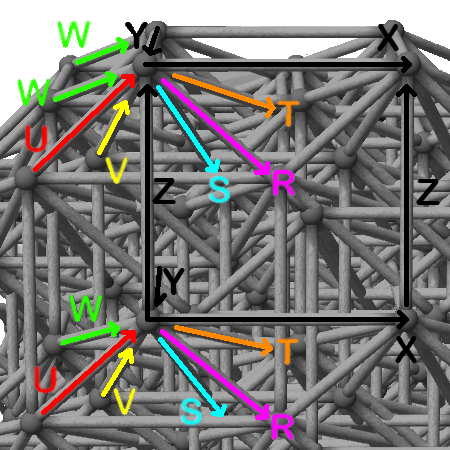## On the Subject of Dimension King

And you thought the nightmare would end with Ultracube and UltraStores...

The module shows a 4D or 5D shape, with the base shape having three to five vertices.

### Getting the info required to solve this module:

First of all, determine the dimension count.

Then, read the sequence of five rotations of the shape. There is a sixth rotation, which is a quintuple rotation, before the sequence restarts.

From the following list of rotation-axis letters, determine the value of each rotation-axis letter:

`X=0, Y=1, Z=2, W=3, V=4, ...`

Now calculate the rotation values Rn, where n is the rotation position starting at 1, 'a' being the value of the first rotation-axis letter, and 'b' being the second:

`Rn = a * {dimensionCount} + b`

Now determine the first two Schläfli numbers, S1 and S2:

The first Schläfli number specifies how many vertices are required to make up a base face.

The second Schläfli number determines how many faces enclose every vertex.

### Coordinate system:

Dimension King uses the N-d coordinate system version 1.0 which was designed to work with up to 9 dimensions. Check the last page for further explanation.

Note: The KTANE bomb factory recently hired new staff and the person that plugs in this module can’t decide where the status light should be, so this module may be rotated. Because the coordinate system is relative to the module, the axes will rotate if the module is rotated.

### Submitting the calculated numbers:

First, click any vertex. The shape will now rotate back to the original position, and then all the vertices will be colored differently.

Compare the colors of the shape’s vertices with the colors in the following table:

Color Value
Red 0
Blue 1
Yellow 2
Green 3
Orange 4
Color Value
Cyan 5
Magenta 6
Lime 7
Key 8
White 9

The lowest value in the table, which is also present on the shape has a value of 0. The next color present on the shape has a value of 1 and so on.

Once the values of the colors are determined, the solution can be submitted.

The following numbers need to be submitted in this order: R1 R2 R3 R4 R5 S1 S2

The first clicked color determines the amount of additional numbers that are going to be entered. Once a whole sequence is entered, the sum of all numbers except for the first one is taken and submitted.

This sum has to be equal to the number that should be inputted. Otherwise a strike will be incurred and the module will reset along with the input.

Once all the numbers are entered correctly, the module is solved.

## N-d coordinate system version 1.0

This coordinate system may also be used by other modules.

The N-d coordinate system is designed to work for a variable number of dimensions, ranging from 3 to 9, hence the N, which is a natural number.To the right you see a figure showing a 9 dimensional representation of this coordinate system, mapped to a 9 dimensional cube. In this special case, every axis points along an edge of the cube.
Every rotation is described by the two axes which enclose the plate that is rotating. If the 9-cube, as shown in the image, were to rotate clockwise then this would be a rotation of the plane enclosed by X and Z. If looking at the bottom left vertex, then it would move along the Z axis and then along the X axis, making this an ZX rotation. If looking at the top right vertex, it would be a -Z-X rotation, which is the same as ZX, as shown below.

### Converting negative rotations to the default notation:

The default notation is made of two axis letters, written uppercase, without any minus signs, e.g. `XZ` or `ZX`, but not `X-Z`, `-XZ`, nor `-Z-X`.

Input Default Notation
+X+Z XZ
-X+Z ZX
+X-Z ZX
-X-Z XZ

The table to the right shows how to convert rotations to the proper notation, which is then used by modules. The basic way of doing it is by swapping the two letters if the number of minus signs is equal to 1, and then removing all minus signs.